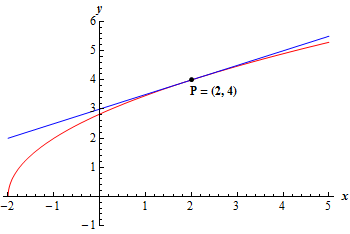Paul's Online Notes
Home / Calculus I / Limits / Tangent Lines and Rates of Change
Show Mobile Notice Show All Notes Hide All Notes
Mobile Notice
You appear to be on a device with a "narrow" screen width (i.e. you are probably on a mobile phone). Due to the nature of the mathematics on this site it is best views in landscape mode. If your device is not in landscape mode many of the equations will run off the side of your device (should be able to scroll to see them) and some of the menu items will be cut off due to the narrow screen width.

### Section 2.1 : Tangent Lines And Rates Of Change

2. For the function $$g\left( x \right) = \sqrt {4x + 8}$$ and the point $$P$$ given by $$x = 2$$ answer each of the following questions.

1. For the points $$Q$$ given by the following values of $$x$$ compute (accurate to at least 8 decimal places) the slope, $${m_{PQ}}$$, of the secant line through points $$P$$ and $$Q$$.
1. 2.5
2. 2.1
3. 2.01
4. 2.001
5. 2.0001
1. 1.5
2. 1.9
3. 1.99
4. 1.999
5. 1.9999
2. Use the information from (a) to estimate the slope of the tangent line to $$g\left( x \right)$$ at $$x = 2$$ and write down the equation of the tangent line.

Show All Solutions Hide All Solutions

a For the points $$Q$$ given by the following values of $$x$$ compute (accurate to at least 8 decimal places) the slope, $${m_{PQ}}$$, of the secant line through points $$P$$ and $$Q$$. Show Solution
1. 2.5
2. 2.1
3. 2.01
4. 2.001
5. 2.0001
1. 1.5
2. 1.9
3. 1.99
4. 1.999
5. 1.9999

The first thing that we need to do is set up the formula for the slope of the secant lines. As discussed in this section this is given by,

${m_{PQ}} = \frac{{g\left( x \right) - g\left( 2 \right)}}{{x - 2}} = \frac{{\sqrt {4x + 8} - 4}}{{x - 2}}$

Now, all we need to do is construct a table of the value of $${m_{PQ}}$$ for the given values of $$x$$. All of the values in the table below are accurate to 8 decimal places.

$$x$$ $${m_{PQ}}$$ $$x$$ $${m_{PQ}}$$
2.5 0.48528137 1.5 0.51668523
2.1 0.49691346 1.9 0.50316468
2.01 0.49968789 1.99 0.50031289
2.001 0.49996875 1.999 0.50003125
2.0001 0.49999688 1.9999 0.50000313

b Use the information from (a) to estimate the slope of the tangent line to $$g\left( x \right)$$ at $$x = 2$$ and write down the equation of the tangent line. Show Solution

From the table of values above we can see that the slope of the secant lines appears to be moving towards a value of 0.5 from both sides of $$x = 2$$ and so we can estimate that the slope of the tangent line is : $$\require{bbox} \bbox[2pt,border:1px solid black]{{m = 0.5 = \frac{1}{2}}}$$.

The equation of the tangent line is then,

$y = g\left( 2 \right) + m\left( {x - 2} \right) = 4 + \frac{1}{2}\left( {x - 2} \right)\hspace{0.5in} \Rightarrow \hspace{0.5in}\,\require{bbox} \bbox[2pt,border:1px solid black]{{y = \frac{1}{2}x + 3}}$

Here is a graph of the function and the tangent line.